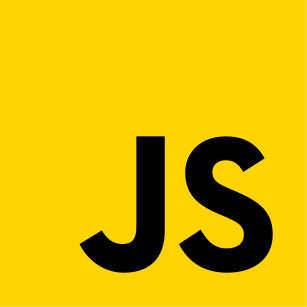Codistwa

# Practice Big O notation and complexity time - Quiz

beginnerfundamentals

## level 1

You've read the guide, now test your knowledge of Big O notation and complexity time! If you have any doubt, you can go back to the guide.### Question 1

What's the time complexity of this code?javascript
1function question(num) {
2  for (i = 1; i <= num; i *= 2) {
3    console.log(i);
4  }
5}
Select...

### Question 2

What will be the number of executions?javascript
1function executions(num) {
2  for (let i = 1; i <= num; i++) {
3    for (let j = 1; j <= num; j++) {
4      for (let k = 1; k <= num; k++) {
5        console.log(i, j, k)
6      }
7    }
8  }
9}
10
11console.log(executions(3));
Select...

### Question 3

Which function is factorial?

1
2
Select...

### Question 4

Which code corresponds to linearithmic complexity?

1javascript
1function response1(num) {
2  for (let i = 0; i < num; i++) {
3    console.log(i);
4  }
5}
6
7console.log(response1(10))
2javascript
1function response2(num) {
2  for (let i = 1; i <= num; i++) {
3    for (let j = 1; j < num; j *= 2) {
4      console.log(i, j)
5    }
6  }
7}
8
9console.log(response2(4));
Select...

### Question 5

How to interpret this comic strip?

Select...